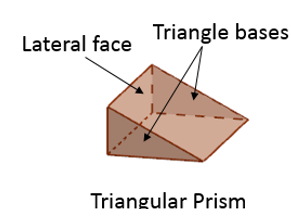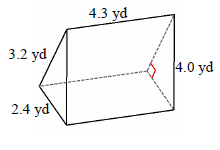# Surface area of a triangular prism

#### Surface Areas and Volumes

117 Lectures 6.5 hours

#### Class 10th - Surface Areas and Volumes

20 Lectures 1 hours

#### Class 10th - Surface Areas and Volumes (Hindi)

10 Lectures 47 mins

In this lesson, we learn how to find the surface area of a triangular prism.

A triangular prism is a prism that has two congruent triangles as its bases connected by three rectangular lateral faces.The surface area of the triangular prism is the sum total of the areas of its bases and its lateral faces.

Formula to find the surface area of a triangular prism

The Surface Area = 2 B + p h

where,

B is the area of the triangular base of prism

p perimeter of the base and

h the height of the prism

Find the surface area of the following triangular prism.### Solution

Step 1:

Area of triangle base A = 17.34 sq ft; height of prism h = 3.3 ft

Step 2:

Surface area of triangular prism = 2A + (a + b + c )h

= 2 × 17.34 + (6.8 + 8.5 + 5.1) × 3.3

= 102 square ft

Find the surface area of the following triangular prism.### Solution

Step 1:

Area of triangle base A = 3.84 sq yd; height of prism h = 4.3 yd

Step 2:

Surface area of triangular prism = 2A + (a + b + c)h

= 2 × 3.84 + (2.4 + 3.2 + 4) × 4.3

= 48.96 square yd